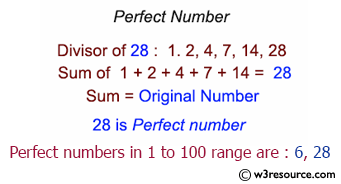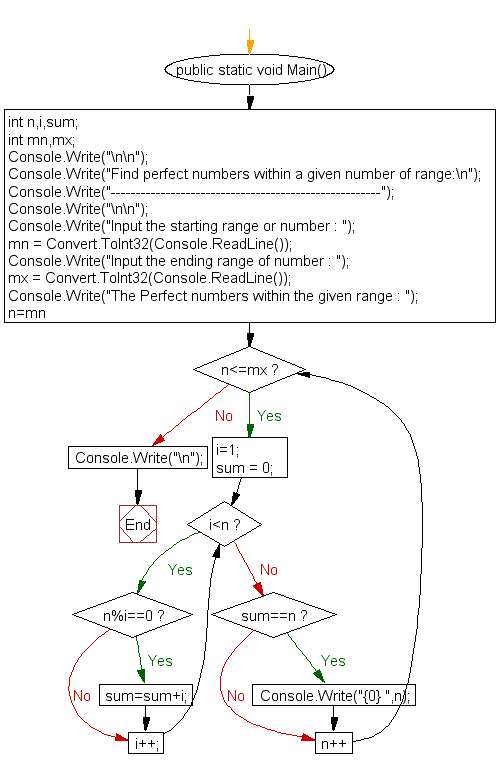﻿ C# - Find perfect numbers within a given range of number# C# Sharp Exercises: Find perfect numbers within a given range of number

## C# Sharp For Loop: Exercise-28 with Solution

Write a C# Sharp Program to find the perfect numbers within a given range of number.

Pictorial Presentation:Sample Solution:

C# Sharp Code:

``````/*Perfect number is a positive number which sum of all positive divisors excluding*/
/*that number is equal to that number. For example 6 is perfect number since divisor of 6 are 1, 2 and 3.*/
/*Sum of its divisor is 1 + 2+ 3 = 6*/
using System;
public class Exercise28
{
public static void Main()
{
int n,i,sum;
int mn,mx;

Console.Write("\n\n");
Console.Write("Find perfect numbers within a given number of range:\n");
Console.Write("------------------------------------------------------");
Console.Write("\n\n");

Console.Write("Input the starting range or number : ");
Console.Write("Input the ending range of number : ");
Console.Write("The Perfect numbers within the given range : ");
for(n=mn;n<=mx;n++)
{
i=1;
sum = 0;
while(i<n)
{
if(n%i==0)
sum=sum+i;
i++;
}
if(sum==n)
Console.Write("{0} ",n);
}
Console.Write("\n");
}
}
```
```

Sample Output:

```Find perfect numbers within a given number of range:
------------------------------------------------------
Input the starting range or number : 1
Input the ending range of number : 20
The Perfect numbers within the given range : 6
```

Flowchart:C# Sharp Code Editor:

Contribute your code and comments through Disqus.

What is the difficulty level of this exercise?

Test your Programming skills with w3resource's quiz.

﻿